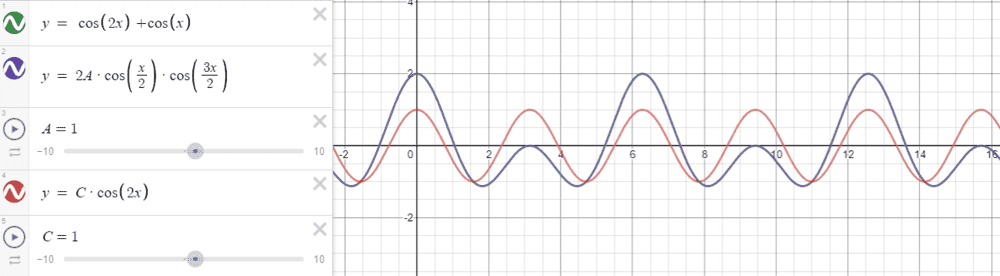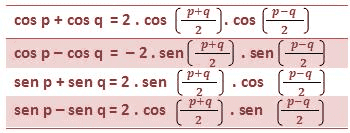# Beats and superposition > doubt about the math

• LCSphysicist
In summary, the superposition of two MHS functions with different frequencies can lead to a periodic function if the frequencies are related to each other by a simple fraction. However, the product of two periodic functions is not necessarily periodic. This is because the sum of two cosines can be equivalent to a product of two cosines with different frequencies, which can lead to a changing amplitude rather than a periodic function.

#### LCSphysicist

Homework Statement
All below
Relevant Equations
All below
Is said that, the superposition of two MHS
X1 = A*cos(c/T1*t)
X2 = A*cos(c/T2*t)

The superposition will be a function which the period will be T in such way that satisfy:
T = n1*T1 = n2*T2

But, if we add X1 and X2 we obtain:

X = 2A*cos(((c/T1) - (c/T2))/2)*cos(((c/T1) + (c/T2))/2)

Why those two equations lead us to differents periods?

LCSphysicist said:
Homework Statement:: All below
Relevant Equations:: All below

Is said that, the superposition of two MHS
X1 = A*cos(c/T1*t)
X2 = A*cos(c/T2*t)

The superposition will be a function which the period will be T in such way that satisfy:
T = n1*T1 = n2*T2

But, if we add X1 and X2 we obtain:

X = 2A*cos(((c/T1) - (c/T2))/2)*cos(((c/T1) + (c/T2))/2)

Why those two equations lead us to differents periods?
The time is missing in your formula. If the formula is written correctly, when is it really periodic and what is that period?

•Delta2
Let say
$$X/A=cos\omega_1 t + cos\omega_2 t$$
LCSphysicist said:
The superposition will be a function which the period will be T in such way that satisfy:
T = n1*T1 = n2*T2
$$\frac{\omega_1 T}{2\pi}=n_1$$
$$\frac{\omega_2 T}{2\pi}=n_2$$
,integers. Estimate
$$\frac{(\omega_1-\omega_2) /2}{2\pi}T=(n_1-n_2)/2$$
$$\frac{(\omega_1+\omega_2) /2}{2\pi}T=(n_1+n_2)/2$$

Last edited:
•LCSphysicist
What is a periodic function? And how is its period defined?
The product of two periodic function need not be periodic.
Consider the functions ##f_1(t)=\cos(t) ## (it is periodic with T1=2pi) and ##f_2(t)= \cos(\pi t)## (period is T2=2) . Is the product ##f_1(t)f_2(t)## =periodic?
You can not say that an f(t) function has two periods.

•Delta2
ehild said:
What is a periodic function? And how is its period defined?
The product of two periodic function need not be periodic.
Consider the functions ##f_1(t)=\cos(t) ## (it is periodic with T1=2pi) and ##f_2(t)= \cos(\pi t)## (period is T2=2) . Is the product ##f_1(t)f_2(t)## =periodic?
You can not say that an f(t) function has two periods.
But in the case that is periodic, yet the formulas lead us to different periods.I agree we can not say f1f2 is periodic, but i am trying to see if it is, so the two formulas would need to be right.

anuttarasammyak said:
Let say
$$X/A=cos\omega_1 t + cos\omega_2 t$$

$$\frac{\omega_1 T}{2\pi}=n_1$$
$$\frac{\omega_2 T}{2\pi}=n_2$$
,integers. Estimate
$$\frac{(\omega_1-\omega_2) /2}{2\pi}T=(n_1-n_2)/2$$
$$\frac{(\omega_1+\omega_2) /2}{2\pi}T=(n_1+n_2)/2$$
I am not sure what you want to say, the two formulas lead us to the right answer??

The sum of two cosines (edit: of equal amplitudes) with different frequencies f1 and f2 is equivalent to a product of two cosines, one with frequency (f1+f2)/2 and the other with frequency (f1-f2)/2. That is how beats appear if the two frequencies are close to each other:the factor with the low frequency appears as periodically changing amplitude of a vibration with the average frequency.
To observe beats, the sum of the two cosines need not be periodic,but it is periodic if nT1=mT2.

Last edited:
•Delta2 and LCSphysicist
Thank you all, now i see my error.
I was doing:

`{Example}

y = cos(x)
y = cos(2x)

T = n1*2*pi = n2*pi (1)

So n1 = 1, n2 = 2 (2)

My error was when i cutted the pi, because i ended with

T = n1*2 = n2 (3)

and instead put the values (2) i found in (1), i was putting in (3)

LCSphysicist said:
I am not sure what you want to say, the two formulas lead us to the right answer??
They are both integers or ...

•LCSphysicist
rude man said:
I think what he want to say is a math implication•Delta2 and Motocross9
I believe your question has been answered, but here is a video I made about beats/superposition of modes for a coupled pendulum, it may help:

•LCSphysicist and rude man
Your video is a great depiction of superposition (linear process) and should significantly help address the confusion between that and mixing (non-linear process).

•Motocross9